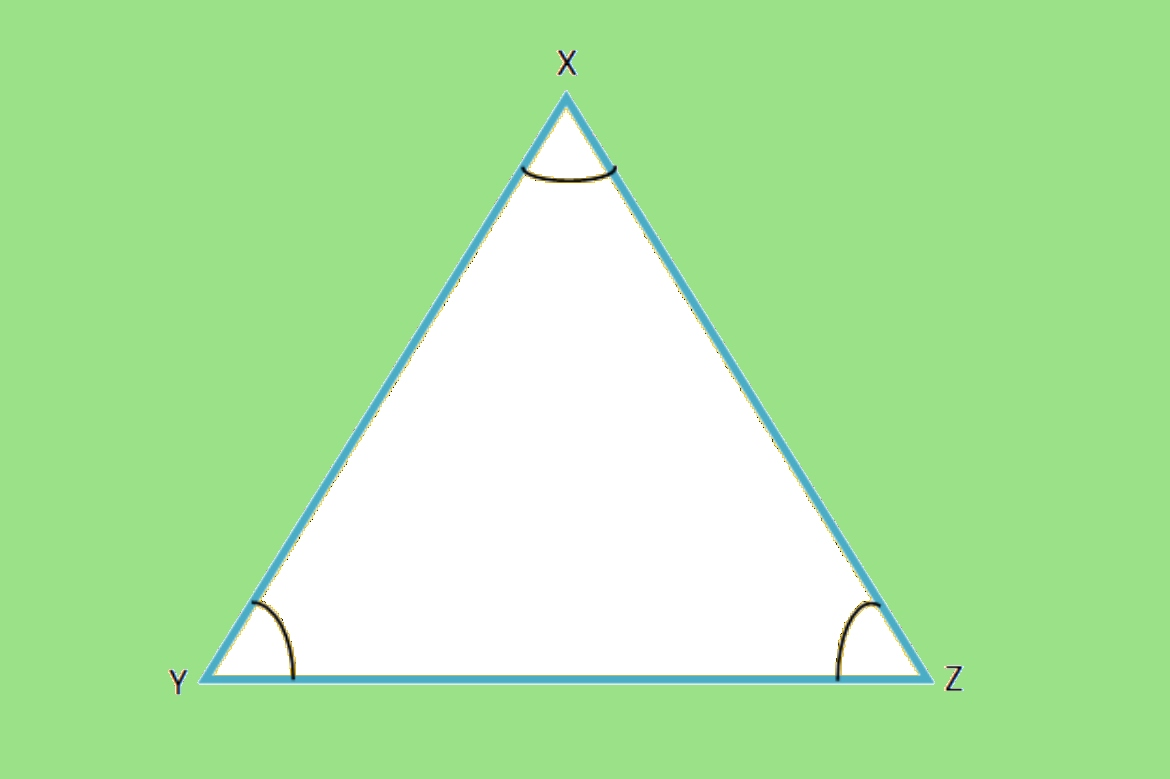# Area Of Equilateral TriangleThe term ‘Equilateral Triangle‘ refers to a triangle with each side equal in length. The measure of all internal angles in an equilateral triangle is 60 degrees. In a two-dimensional plane, area of an equilateral triangle is the amount of space it takes up. It is always measured in square units. As a result, an equilateral triangle is a typical example of an isosceles triangle in which all three sides are equal.

## Area Of A Triangle

Basically, a triangle is a three-sided polygon. It has three vertices. Angles on the interior of a triangle add up to 180°, while angles on the exterior are 360°. Triangles are of three types based on the length of their sides, isosceles triangle, equilateral triangle, and a right-angled triangle. Thus, the area of a triangle is equal to the total area occupied by its three sides. The area of the triangle is half of the base and height of the triangle.

## What Do You Mean By The Area Of An Equilateral Triangle?

The area of a triangle is how much space it occupies in a two-dimensional plane. The equilateral triangle is a three-sided closed shape with all three sides equal and an internal angle of 60 °. It is a special case of the isosceles triangle in which the third side is also equal. If △ ABC is an equilateral triangle then AB=BC=CA and ∠A = ∠B = ∠C= 60°

## Area of an Equilateral Triangle Formula

Triangles with three equal sides and 60° angles on all sides are known as equilateral triangles. When a perpendicular bisector line is traced through the vertex of an equilateral, two right triangles are created. By using the Pythagorean theorem and the height of right-angled triangles you can find the missing side lengths of an equilateral triangle.
The area of an equilateral triangle can be calculated using the formula given below:
Area of Equilateral Triangle (A) = √3/4 a2

Where a = length of sides

## Area Of Equilateral Triangle Using Heron’s Formula

We can use Heron’s formula to find the area of any triangle, whether it is scalene, isosceles or equilateral, provided the sides of the triangle are known.
Consider a triangle ABC whose sides are a, b, and c, respectively. An area of a triangle can therefore be calculated as follows:
Area =√((s(s-a)(s-b)(s-c)))
S is the semi-perimeter and a,b and c are the length of the sides
If it is an equilateral triangle
Area=√(s(s-a)2^ )( since all sides are equal)
s= (a+b+c)/2= (a+a+a)/2
Therefore, s= 3a/2

### Examples

Example 1. Find the Area of an Equilateral Triangle each of whose sides are 8 cm.

Solution:  Given each side of an equilateral triangle = a = 8 cm.

The formula for area of an equilateral triangle =  a2

=  * 8 * 8  (cm)2

= 16     cm2

Example 2. Find the length of the side  of an Equilateral Triangle with Area 9     cm2

Solution: We know the area of equilateral triangle =  a2

=  a2

Area of Equilateral Triangle is given as  9     cm2

∴ 9    =    a2

a2    =  36

∴ a     = 6

∴ The length of the side is 6 cm.

Example 3: Calculate the area of a triangle with a base of 12 inches and a height of 7 inches.

Solution:

Area of a triangle =  * base(b) * height(h)

A =   × b × h sq.units

⇒ A = (½) × (12 in) × (7 in)

⇒ A = (½) × (84) ( in)2

⇒ A = 42 in2

∴ The area of a triangle is  42 in2